# T-distribution Calculator - Online

Below you can find a T-distribution Calculator. The shape of the Student's t-distribution is determined by the degrees of freedom. Its shape changes as the degrees of freedom increases.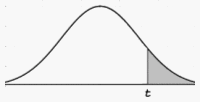Student's t-distribution curve

The shaded area represents the right-tail value for t.

The right-tail value is approximated by using the following formula: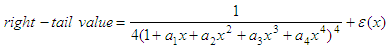where

a1 = 0.196854
a2 = 0.115194
a3 = 0.000344
a4 = 0.019527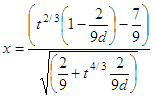d = degrees of freedom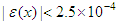### Example:

Determine the right-tail value when t = 2.9 and degrees of freedom dof = 16.

Enter T-value = 2.9
Enter Degrees of freedom = 16

and you get from the calculator

Right-tail value =  0.0051
Left-tail value = 0.9949

This is an image showing another example of how you can use the calculator: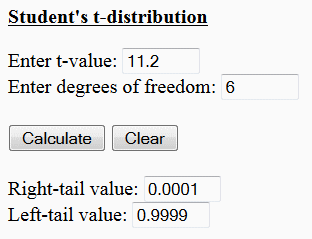Please, enable JavaScript codes on this page, otherwise the calculator will not work.Student's t-distribution Enter t-value: Enter degrees of freedom: Right-tail value: Left-tail value:

From 'T Distribution Calculator' to home

From 'T Distribution Calculator' to Free Online Calculators

 Top T-Distribution code in Matlab